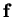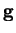Next: Previous hybrid models Up: Nonlinear state-space models Previous: Nonlinear factor analysis   Contents

## Learning algorithms

The most important NSSM learning algorithm for this work is the one by Dr. Harri Valpola [58,60]. It uses MLP networks to model the nonlinearities and ensemble learning to optimise the model. It is discussed in detail in Sections 5.2 and 6.2 and it will therefore be skipped for now.

Even though it is not exactly a learning algorithm for complete NSSMs, the extended Kalman filter (EKF) is an important building block for many such algorithms. The EKF extends standard Kalman filtering for nonlinear models. The nonlinear functionsandof Equation (4.13) must be known in advance. The algorithm works by linearising the functions about the estimated posterior mean. The posterior probability of the state variables is evaluated with a forward-backward type iteration by assuming the posterior of each sample to be Gaussian [42,28,32].

Briegel and Tresp  present an NSSM that uses MLP networks as the model of the nonlinearities. The learning algorithm is based on Monte-Carlo generalised EM algorithm, i.e. an EM algorithm with stochastic estimates for the conditional posteriors at different steps. The Monte-Carlo E-step is further optimised by generating the samples of the hidden states from an approximate Gaussian distribution instead of the true posterior. The approximate posterior is found using either the EKF or an alternative similar method.

Roweis and Ghahramani [51,19] use RBF networks to model the nonlinearities. They use standard EM algorithm with EKF for the approximate E-step. The parameterisation of the RBF network allows an exact M-step for some of the parameters. All the parameters of the network can not, however, be adapted this way.Next: Previous hybrid models Up: Nonlinear state-space models Previous: Nonlinear factor analysis   Contents
Antti Honkela 2001-05-30